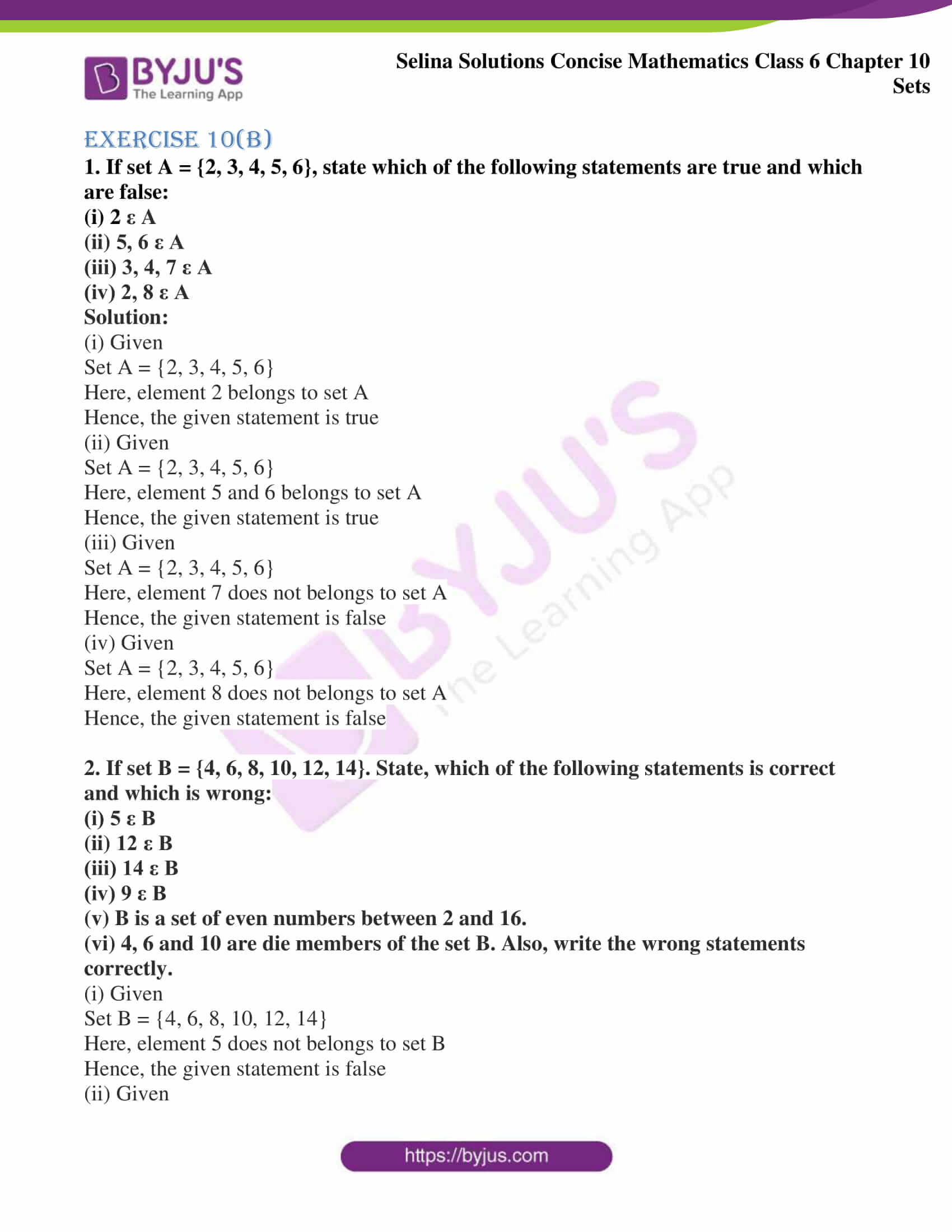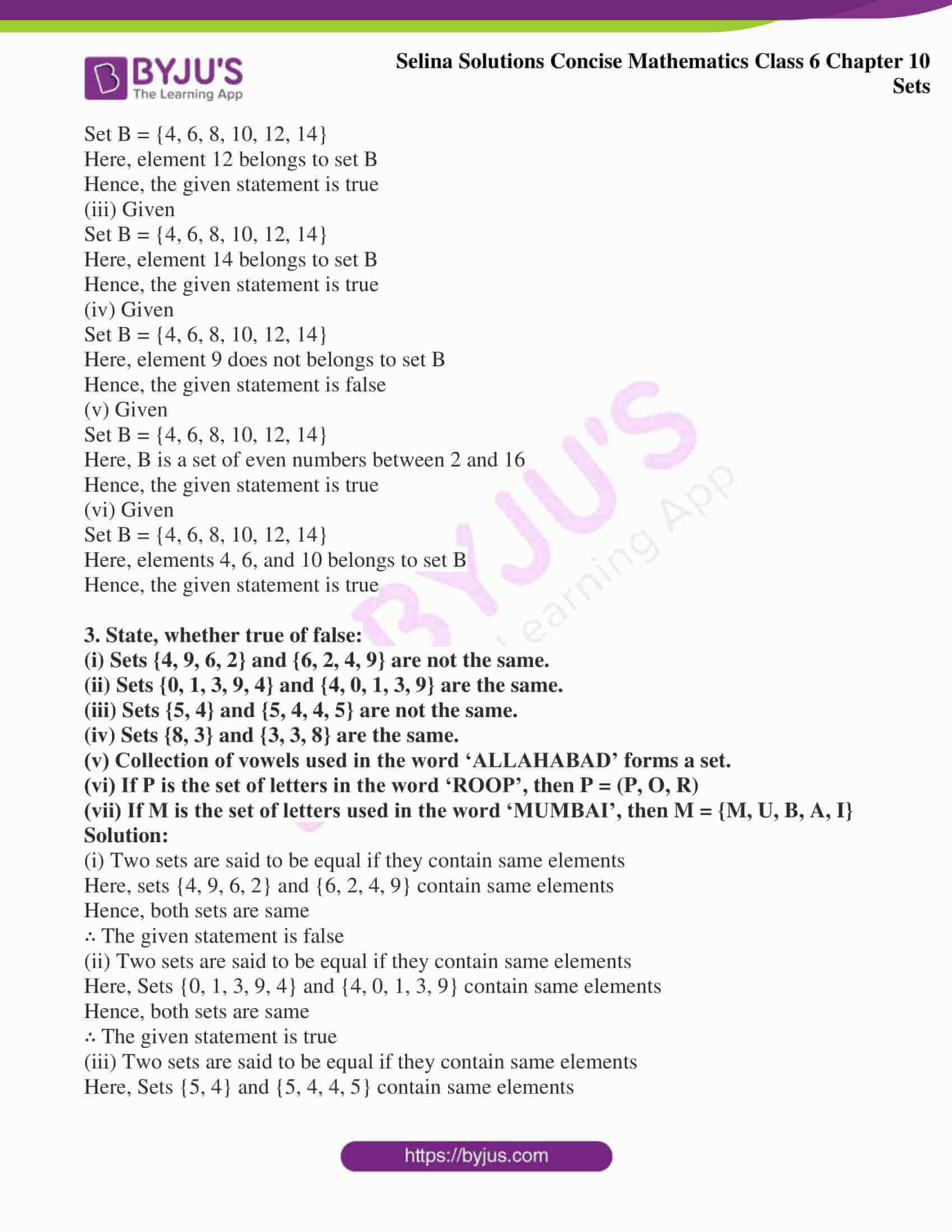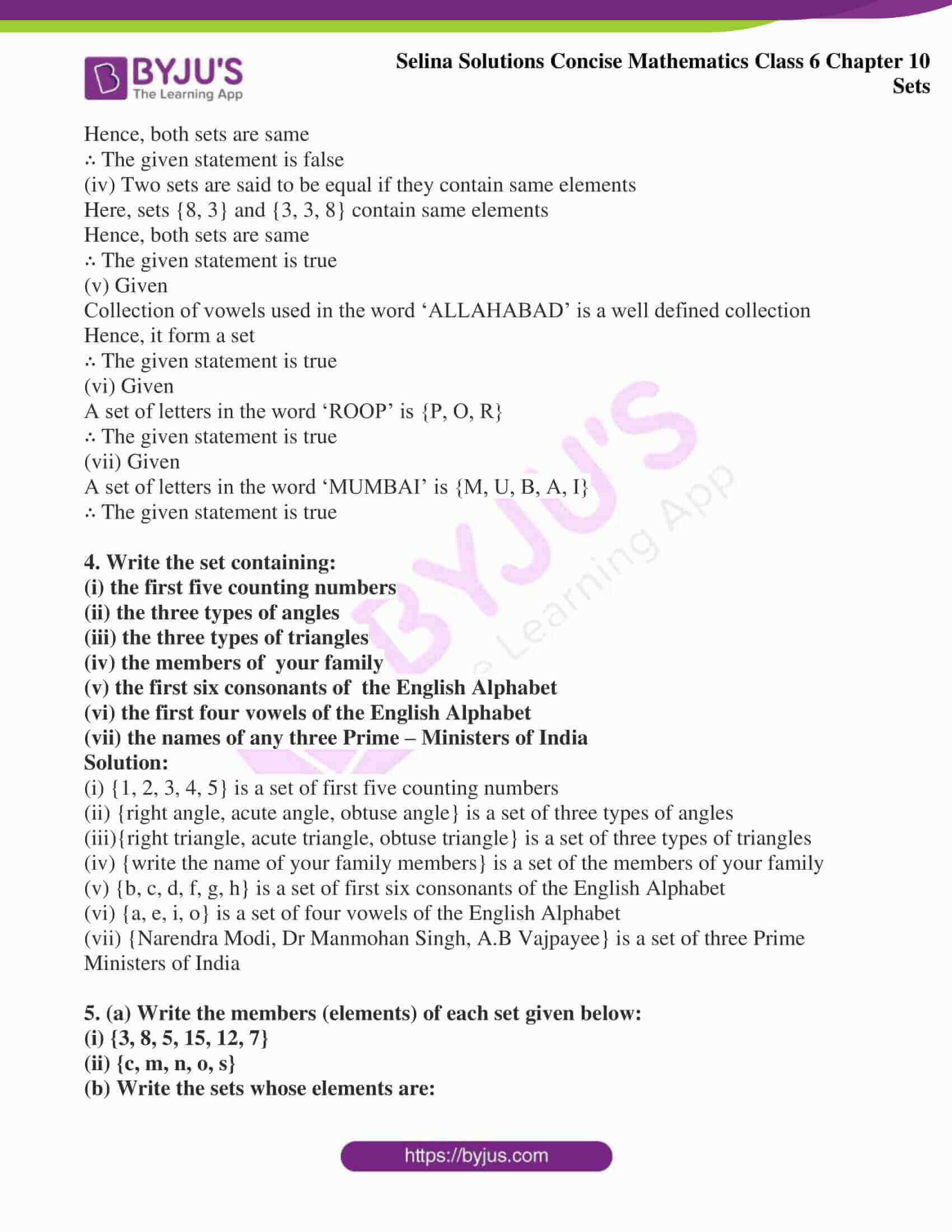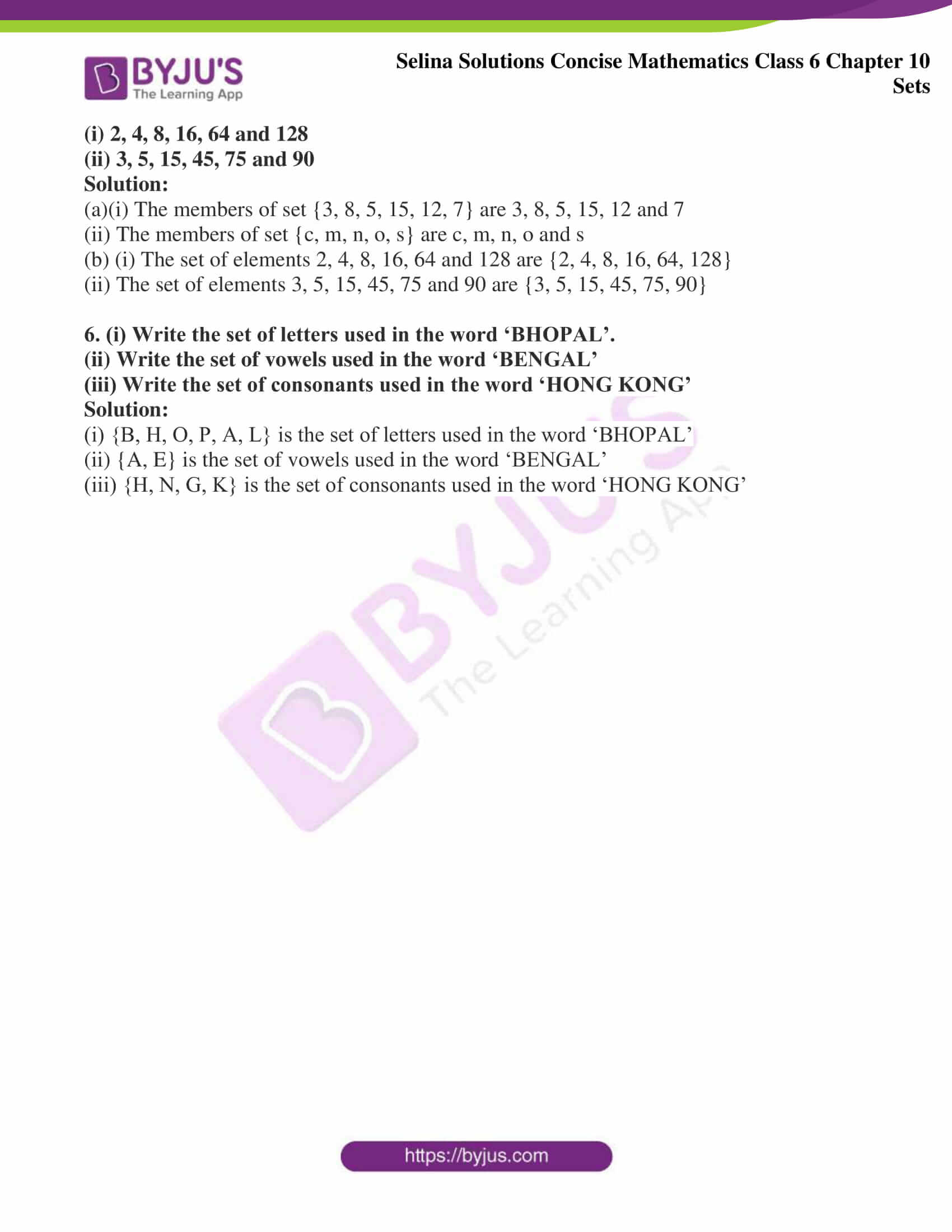# Selina Solutions Concise Mathematics Class 6 Chapter 10: Sets Exercise 10 (B)

Selina Solutions Concise Mathematics Class 6 Chapter 10: Sets Exercise 10(B) provides accurate answers to each and every question of the textbook covered under this exercise. These Solutions are curated by the faculty at BYJU’S, in accordance with the current ICSE exams with the desire to help students in exam preparation. Practising Selina Solutions systematically on a daily basis helps students to perform well in exams. Avail Selina Solutions Concise Mathematics Class 6 Chapter 10 Sets Exercise 10(B) in PDF, from the given links below

## Selina Solutions Concise Mathematics Class 6 Chapter 10: Sets Exercise 10(B) Download PDF### Access other exercises of Selina Solutions Concise Mathematics Class 6 Chapter 10: Sets

Exercise 10(A) Solutions

Exercise 10(C) Solutions

Exercise 10(D) Solutions

Exercise 10(E) Solutions

### Access Selina Solutions Concise Mathematics Class 6 Chapter 10: Sets Exercise 10(B)

#### Exercise 10(B)

1. If set A = {2, 3, 4, 5, 6}, state which of the following statements are true and which are false:

(i) 2 ε A

(ii) 5, 6 ε A

(iii) 3, 4, 7 ε A

(iv) 2, 8 ε A

Solution:

(i) Given

Set A = {2, 3, 4, 5, 6}

Here, element 2 belongs to set A

Hence, the given statement is true

(ii) Given

Set A = {2, 3, 4, 5, 6}

Here, element 5 and 6 belongs to set A

Hence, the given statement is true

(iii) Given

Set A = {2, 3, 4, 5, 6}

Here, element 7 does not belongs to set A

Hence, the given statement is false

(iv) Given

Set A = {2, 3, 4, 5, 6}

Here, element 8 does not belongs to set A

Hence, the given statement is false

2. If set B = {4, 6, 8, 10, 12, 14}. State, which of the following statements is correct and which is wrong:

(i) 5 ε B

(ii) 12 ε B

(iii) 14 ε B

(iv) 9 ε B

(v) B is a set of even numbers between 2 and 16.

(vi) 4, 6 and 10 are die members of the set B. Also, write the wrong statements correctly.

(i) Given

Set B = {4, 6, 8, 10, 12, 14}

Here, element 5 does not belongs to set B

Hence, the given statement is false

(ii) Given

Set B = {4, 6, 8, 10, 12, 14}

Here, element 12 belongs to set B

Hence, the given statement is true

(iii) Given

Set B = {4, 6, 8, 10, 12, 14}

Here, element 14 belongs to set B

Hence, the given statement is true

(iv) Given

Set B = {4, 6, 8, 10, 12, 14}

Here, element 9 does not belongs to set B

Hence, the given statement is false

(v) Given

Set B = {4, 6, 8, 10, 12, 14}

Here, B is a set of even numbers between 2 and 16

Hence, the given statement is true

(vi) Given

Set B = {4, 6, 8, 10, 12, 14}

Here, elements 4, 6, and 10 belongs to set B

Hence, the given statement is true

3. State, whether true or false:

(i) Sets {4, 9, 6, 2} and {6, 2, 4, 9} are not the same.

(ii) Sets {0, 1, 3, 9, 4} and {4, 0, 1, 3, 9} are the same.

(iii) Sets {5, 4} and {5, 4, 4, 5} are not the same.

(iv) Sets {8, 3} and {3, 3, 8} are the same.

(v) Collection of vowels used in the word ‘ALLAHABAD’ forms a set.

(vi) If P is the set of letters in the word ‘ROOP’, then P = (P, O, R)

(vii) If M is the set of letters used in the word ‘MUMBAI’, then M = {M, U, B, A, I}

Solution:

(i) Two sets are said to be equal if they contain the same elements

Here, sets {4, 9, 6, 2} and {6, 2, 4, 9} contain same elements

Hence, both sets are same

∴ The given statement is false

(ii) Two sets are said to be equal if they contain same elements

Here, Sets {0, 1, 3, 9, 4} and {4, 0, 1, 3, 9} contain same elements

Hence, both sets are same

∴ The given statement is true

(iii) Two sets are said to be equal if they contain same elements

Here, Sets {5, 4} and {5, 4, 4, 5} contain same elements

Hence, both sets are same

∴ The given statement is false

(iv) Two sets are said to be equal if they contain same elements

Here, sets {8, 3} and {3, 3, 8} contain same elements

Hence, both sets are same

∴ The given statement is true

(v) Given

Collection of vowels used in the word ‘ALLAHABAD’ is a well defined collection

Hence, it form a set

∴ The given statement is true

(vi) Given

A set of letters in the word ‘ROOP’ is {P, O, R}

∴ The given statement is true

(vii) Given

A set of letters in the word ‘MUMBAI’ is {M, U, B, A, I}

∴ The given statement is true

4. Write the set containing:

(i) the first five counting numbers

(ii) the three types of angles

(iii) the three types of triangles

(iv) the members of your family

(v) the first six consonants of the English Alphabet

(vi) the first four vowels of the English Alphabet

(vii) the names of any three Prime – Ministers of India

Solution:

(i) {1, 2, 3, 4, 5} is a set of first five counting numbers

(ii) {right angle, acute angle, obtuse angle} is a set of three types of angles

(iii){right triangle, acute triangle, obtuse triangle} is a set of three types of triangles

(iv) {write the name of your family members} is a set of the members of your family

(v) {b, c, d, f, g, h} is a set of first six consonants of the English Alphabet

(vi) {a, e, i, o} is a set of four vowels of the English Alphabet

(vii) {Narendra Modi, Dr Manmohan Singh, A.B Vajpayee} is a set of three Prime Ministers of India

5. (a) Write the members (elements) of each set given below:

(i) {3, 8, 5, 15, 12, 7}

(ii) {c, m, n, o, s}

(b) Write the sets whose elements are:

(i) 2, 4, 8, 16, 64 and 128

(ii) 3, 5, 15, 45, 75 and 90

Solution:

(a)(i) The members of set {3, 8, 5, 15, 12, 7} are 3, 8, 5, 15, 12 and 7

(ii) The members of set {c, m, n, o, s} are c, m, n, o and s

(b) (i) The set of elements 2, 4, 8, 16, 64 and 128 are {2, 4, 8, 16, 64, 128}

(ii) The set of elements 3, 5, 15, 45, 75 and 90 are {3, 5, 15, 45, 75, 90}

6. (i) Write the set of letters used in the word ‘BHOPAL’.

(ii) Write the set of vowels used in the word ‘BENGAL’

(iii) Write the set of consonants used in the word ‘HONG KONG’

Solution:

(i) {B, H, O, P, A, L} is the set of letters used in the word ‘BHOPAL’

(ii) {A, E} is the set of vowels used in the word ‘BENGAL’

(iii) {H, N, G, K} is the set of consonants used in the word ‘HONG KONG’